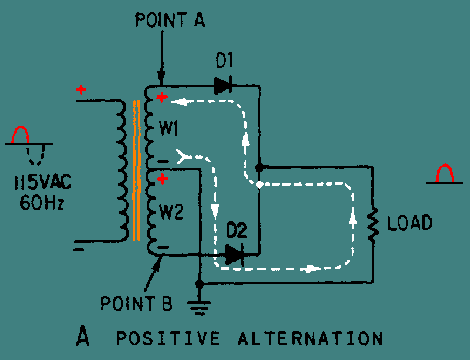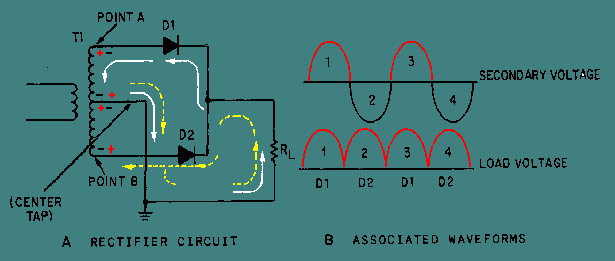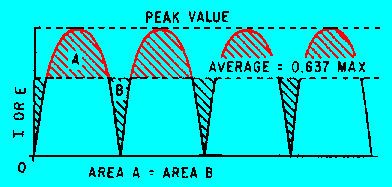Custom SearchThe Conventional Full-Wave Rectifier A full-wave rectifier is a device that has two or more diodes arranged so that load current flows in the same direction during each half cycle of the ac supply. A diagram of a simple full-wave rectifier is shown in figure 4-5. The transformer supplies the source voltage for two diode rectifiers, D1 and D2. This power transformer has a center-tapped, high-voltage secondary winding that is divided into two equal parts (W1 and W2). W1 provides the source voltage for D1, and W2 provides the source voltage for D2. The connections to the diodes are arranged so that the diodes conduct on alternate half cycles. During one alternation of the secondary voltage, the polarities are as shown in view A. The source for D2 is the voltage induced into the lower half of the secondary winding of the transformer (W2). At the specific instant of time shown in the figure, the anode voltage on D2 is negative, and D2 cannot conduct. Throughout the period of time during which the anode of D2 is negative, the anode of D1 is positive. Since the anode of D1 is positive, it conducts, causing current to flow through the load resistor in the direction shown by the arrow. Figure 4-5A. - Full-wave rectifier. POSITIVE ALTERNATIONView B shows the next half cycle of secondary voltage. Now the polarities across W1 and W2 are reversed. During this alternation, the anode of D1 is driven negative and D1 cannot conduct. For the period of time that the anode of D1 is negative, the anode of D2 is positive, permitting D2 to conduct. Notice that the anode current of D2 passes through the load resistor in the same direction as the current of D1 did. In this circuit arrangement, a pulse of load current flows during each alternation of the input cycle. Since both alternations of the input voltage cycle are used, the circuit is called a FULL-WAVE RECTIFIER. Figure 4-5B. - Full-wave rectifier. NEGATIVE ALTERNATIONNow that you have a basic understanding of how a full-wave rectifier works, let's cover in detail a practical full-wave rectifier and its waveforms. A Practical Full-Wave Rectifier A practical full-wave rectifier circuit is shown in view A of figure 4-6. It uses two diodes (D1 and D2) and a center-tapped transformer (T1). When the center tap is grounded, the voltages at the opposite ends of the secondary windings are 180 degrees out of phase with each other. Thus, when the voltage at point A is positive with respect to ground, the voltage at point B is negative with respect to ground. Let's examine the operation of the circuit during one complete cycle. Figure 4-6. - Practical full-wave rectifier.During the first half cycle (indicated by the solid arrows), the anode of D1 is positive with respect to ground and the anode of D2 is negative. As shown, current flows from ground (center tap), up through the load resistor (RL), through diode D1 to point A. In the transformer, current flows from point A, through the upper winding, and back to ground (center tap). When D1 conducts, it acts like a closed switch so that the positive half cycle is felt across the load (RL). During the second half cycle (indicated by the dotted lines), the polarity of the applied voltage has reversed. Now the anode of D2 is positive with respect to ground and the anode of D1 is negative. Now only D2 can conduct. Current now flows, as shown, from ground (center tap), up through the load resistor (RL), through diode D2 to point B of T1. In the transformer, current flows from point B up through the lower windings and back to ground (center tap). Notice that the current flows across the load resistor (RL) in the same direction for both halves of the input cycle. View B represents the output waveform from the full-wave rectifier. The waveform consists of two pulses of current (or voltage) for each cycle of input voltage. The ripple frequency at the output of the full-wave rectifier is therefore twice the line frequency. The higher frequency at the output of a full-wave rectifier offers a distinct advantage: Because of the higher ripple frequency, the output is closely approximate to pure dc. The higher frequency also makes filtering much easier than it is for the output of the half-wave rectifier. In terms of peak value, the average value of current and voltage at the output of the full-wave rectifier is twice as great as that at the output of the half-wave rectifier. The relationship between the peak value and the average value is illustrated in figure 4-7. Since the output waveform is essentially a sine wave with both alternations at the same polarity, the average current or voltage is 63.7 percent (or 0.637) of the peak current or voltage. Figure 4-7. - Peak and average values for a full-wave rectifier.As an equation: Where: Emax = The peak value of the load voltage pulse Eavg = 0.637 X Emax (the average load voltage) Imax = The peak value of the load current pulse Iavg = 0.637 X Imax (the average load current) Example: The total voltage across the high-voltage secondary of a transformer used to supply a full-wave rectifier is 300 volts. Find the average load voltage (ignore the drop across the diode). Solution: Since the total secondary voltage (ES) is 300 volts, each diode is supplied one-half of this value, or 150 volts. Because the secondary voltage is an rms value, the peak load voltage is: Emax = 1.414 X ES Emax = 1.414 X 150 Emax = 212 volts The average load voltage is: Eavg = 0.637 X Emax Eavg = 0.637 X 212 Eavg = 135 volts NOTE: If you have problems with this equation, review the portion of NEETS, module 2, that pertain to this subject. As you may recall from your past studies in electricity, every circuit has advantages and disadvantages. The full-wave rectifier is no exception. In studying the full-wave rectifier, you may have found that by doubling the output frequency, the average voltage has doubled, and the resulting signal is much easier to filter because of the high ripple frequency. The only disadvantage is that the peak voltage in the full-wave rectifier is only half the peak voltage in the half-wave rectifier. This is because the secondary of the power transformer in the full-wave rectifier is center tapped; therefore, only half the source voltage goes to each diode. Fortunately, there is a rectifier which produces the same peak voltage as a half-wave rectifier and the same ripple frequency as a full-wave rectifier. This circuit, known as the BRIDGE RECTIFIER, will be the subject of our next discussion. Q.8 What was the major factor that led to the development of the full-wave rectifier?Q.9 What is the ripple frequency of a full-wave rectifier with an input frequency of 60 Hz?Q.10 What is the average voltage (Eavg) Output of a full-wave rectifier with an output of 100 volts peak?Privacy Statement - Copyright Information. - Contact Us